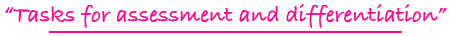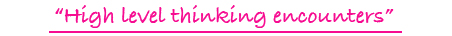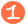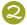•••Tiling Task Card Packets include 20 unique task cards on card stock, 3 backdrop cards, tracking sheets, suggestions and answer sheets.  These are contained in a printed manila envelope for convenient storing.  A set of number tiles 0 - 9 is  needed for each task card.

1 - 3

### VIEWING ADDITION & SUBTRACTION TILES

seeing addition and subtraction representations with black and white circles and writing horizontal addition and subtraction equations

Challenge Level:\$15.00

1 - 3

### VIEWING ADDITION & SUBTRACTION TILES DIGITAL

seeing addition and subtraction representations with black and white circles and writing horizontal addition and subtraction equations

Challenge Level:DIGITAL FORMAT\$10.00

1 - 3

### VIEWING ADDITION & SUBTRACTION TILES

seeing addition and subtraction representations with black and white circles and writing horizontal addition and subtraction equations

Challenge Level:PDF FORMAT
(You will receive both color and black and white files for printing.)\$10.00

5 - 8

### VISUALIZING TILES

dealing with representational models for common fractions, percent, ratio, and square numbers

Challenge Level:\$15.00

5 - 8

### WATCH THE ORDER EQUATION TILES

forming balanced equations plus following the rules for the order of operations; equations may balance to a positive or negative whole number or to a common fraction

Challenge Level:\$15.00

4 - 8

### WAY TO SAY TILES

forming the identified number using addition, subtraction, multiplication, and division

Challenge Level:\$15.00

4 - 8

### WAY TO SAY TILES DIGITAL

forming the identified number using addition, subtraction, multiplication, and division

Challenge Level:DIGITAL FORMAT\$10.00

4 - 8

### WAY TO SAY TILES

forming the identified number using addition, subtraction, multiplication, and division

Challenge Level:PDF FORMAT
(You will receive both color and black and white files for printing.)\$10.00

2 - 5

### X = FUN TILES

adding and subtracting of single-digit and two-digit numbers set up as if...then...functions

Challenge Level:\$15.00

2 - 5

### X = FUN TILES DIGITAL

adding and subtracting of single-digit and two-digit numbers set up as if...then...functions

Challenge Level:DIGITAL FORMAT\$10.00

2 - 5

### X = FUN TILES

adding and subtracting of single-digit and two-digit numbers set up as if...then...functions

Challenge Level:PDF FORMAT
(You will receive both color and black and white files for printing.)\$10.00

1 - 3

### X PICTURE X TILES

counting to show multiplication facts in pictorial arrays; products to 21

Challenge Level:\$15.00